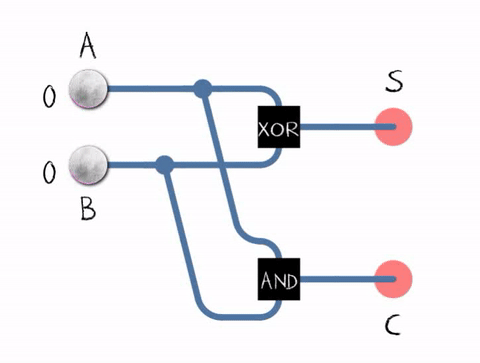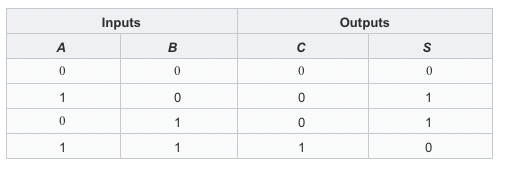Of course you can just return a + b to get accepted. But Can you challenge not do it like that?(You should not use + or any arithmetic operators.)

I encountered such a problem when I did the algorithm question today.

click me to vist

Although it’s not too difficult, this question is quite interesting.

# Problem

## Description

Write a function that add two numbers A and B.

Are a and b both `32-bit` integers?

• Yes.

Can I use bit operation?

• Sure you can.

## challenge

Of course you can just return a + b to get accepted. But Can you challenge not do it like that?(You should not use `+` or any arithmetic operators.)

If we can’t use `+` or any arithmetic operators, we can only use bit operation.

So, we need to understand how the half adder works.

The half adder adds two single binary digits A and B. It has two outputs, sum (S) and carry (C). The carry signal represents an overflow into the next digit of a multi-digit addition. The value of the sum is 2C + S. The simplest half-adder design, pictured on the right, incorporates an XOR gate for S and an AND gate for C.Here is the Half adder in action:The Boolean logic for the sum (in this case S) will be A′B + AB′ whereas for the carry (C) will be AB. With the addition of an OR gate to combine their carry outputs, two half adders can be combined to make a full adder. The half adder adds two input bits and generates a carry and sum, which are the two outputs of a half adder. The input variables of a half adder are called the augend and addend bits. The output variables are the sum and carry. The truth table for the half adder is:## Conversion

Before beginning, let’s divide the decimal A=9 and B=7 into binary A=1001, B=0111.

1. First calculate the number of digits (not carry):

1001 + 0111 = 1110
The result is the same as A^B (A XOR B).

2. Then get the carry.

If 1001 + 0111 do the carry, the carry will occur only when 1 + 1 is there.So, how do we get the carry signal? 1 + 0, 0 + 0, 0 + 1 will not have a carry signal, it satisfies the truth table of phase and &. We can also see directly from the inside of the half adder , S = A ^ B, C = A & B.

3. Plus the carry.

We find that the carry signal appears at 1 + 1 (1&1 = 1), and the previous bit needs to be entered. That is, C can be further expressed as C = (A & B) << 1.

4. repeat and repeat.

Since if the previous bit 0+1 plus the carry symbol 1, it will also be carried. It is necessary to repeat the above process continuously.

# Code

Here I will use the Swift.

Title: Write a function that add two numbers A and B

Author: Tuski

Published: 02/27/2019 - 20:44:26

Updated: 02/28/2019 - 16:41:46